# Hamiltonian simulatioN## Background

The Hamiltonian Simulation problem describes the evolution of quantum systems, such as molecules and solid state systems, by solving the Schrodinger equation. Quantum computers enable the simulation in a scalable manner, as described in [Lloyd96]. The most notable algorithm is the Trotterization-based product formula.

## The Problem

Generate a circuit, using no more than 10 qubits, that approximates the unitary $e^{-iH}$ where $H$ is the qubit hamiltonian of a LiH (lithium hydride) molecule. The LiH Hamiltonian is composed of 276 Pauli strings, and can be found HERE. The approximation error is defined in the next section, and should be less than 0.1. The circuit should be composed of the CX and single qubit gates only.

## Approximation Error

The “distance” between the approximated circuit and the real unitary operator is defined by the operator norm of the difference between the two operators

$error = \|U_{circuit} - e^{-iH}\|$.

The operator norm is defined as the maximal eigenvalue of the operator $\|A\| = max {|\lambda_i|}$ where $\lambda_i$ are the eigenvalues of $A$.

This error describes the worst inconsistency between the two operators for all possible input states.

Note that for unitary operators the error is bounded between 0 and 2.

## Metric

The winning solution will be the circuit with the minimal depth for which the error defined above is less then 0.1.

## Troterrization Algorithm

In this section we are going to describe the most common algorithm - Troterrization based product formula.
The algorithm relays on the Lie-Trotter formula

$$e^{-i(H_1+H_2+...+H_l)t}= \lim_{n\to\infty} (e^{-\frac{iH_1t}{n}}e^{-\frac{iH_2t}{n}}...e^{-\frac{iH_lt}{n}}) ^n.$$

This formula is especially useful in the context of quantum simulations since generally the Hamiltonian is given by a sum of Pauli terms, and a single Pauli term can be exponentiated as presented.

For a Pauli Z Hamiltonian, the unitary $e^{-iZZZt}$ can be presented using the following equivalent circuits: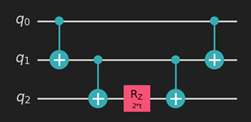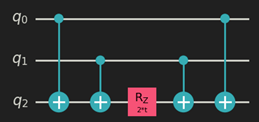An exponentiation of a general Pauli term (consist of I, X, Y, Z Pauli matrices), such as $e^{-iXZXt}$ is composed from $e^{-iZZZt}$ with basis change via single-qubit gates: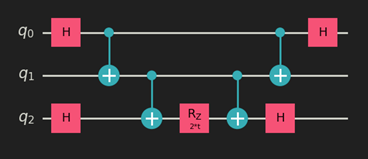Next, we will observe a possibility to optimize the circuit for multi-term Hamiltonian. Note that for a multi-term Hamiltonian such as $H = H_1 + H_2$ the ordering of the terms within a single repetition of the trotter decomposition is irrelevant, i.e

$$e^{-i(H_1 + H_2)t} =\lim_{n\to \infty} (e^{-\frac{iH_1t}{n}}e^{-\frac{iH_2t}n}) = \lim_{n\to \infty} (e^{-\frac{iH_2t}{n}}e^{-\frac{iH_1t}{n}})$$

even if $H_1$ and $H_2$ are not commutative.

These schemes allow compiling of shorter circuits. For example, consider the Hamiltonian $H = ZZZ + ZZ$. Naively the single repetition would compile to: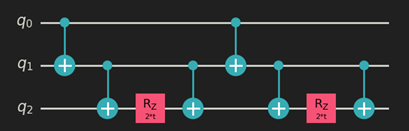Using a different implementation for single term evolution: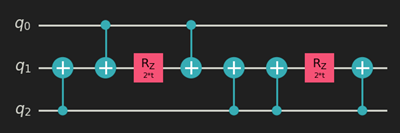some CX gates cancel out to get: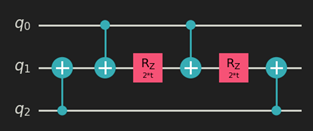## Questions?

Post your questions or see what others have asked on our Competition support site.We look forward to reviewing your solution. Here are some things to know:You are welcome to submit multiple solutions. If you submit something today, and find an improvement later on, you're welcome to submit the new solution as well.Each problem requires slightly different information. Please make sure you carefully review the problem instructions.The description of the approach is very helpful to the judges in understanding your work. Please include as much detail as you wish. We're interested in how you approached this problem, how your solution works, how long it took you to develop it, and whether there were particular problems that you overcame. We'd love to hear as much as you want to tell us. You can submit this in the 'description' field or include it as the 'Additional file (optional)' attachment.The QASM code is how we can verify the 2-qubit gate count and/or circuit depth. It should include only CX and single-qubit unitary gates. This note on our Discourse support site might help.If you created the circuit in something other than QASM, please submit the source code as well.You will receive an email confirming the submission within a few minutes.
Submissions are closed. the results are here. Thank you!
0
DAYS
:
0
Hours
:
0
Minutes
:
0
Seconds
Oops! Something went wrong while submitting the form.
Submission
*QASM Code